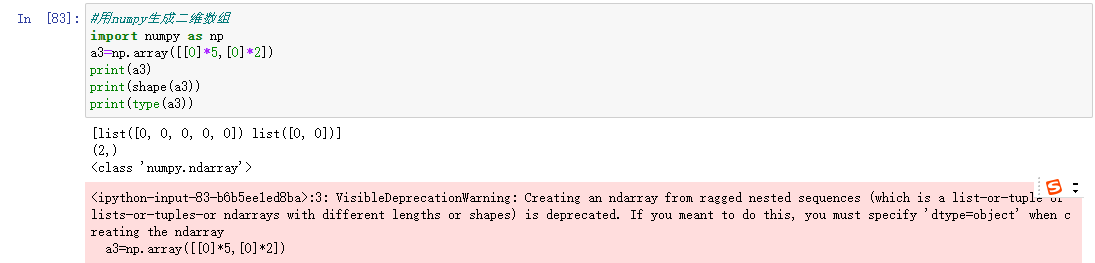• python生成二维数组
2020-08-29 10:22:31
m = [*m for i in range(n)]

更多相关内容
• 下面小编就为大家分享一篇Python实现二维数组输出为图片，具有很好的参考价值，希望对大家有所帮助。一起跟随小编过来看看吧
• 今天小编就为大家分享一篇python将三维数组展开成二维数组的实现，具有很好的参考价值，希望对大家有所帮助。一起跟随小编过来看看吧
• 做leetcode时，想用python生成二维数组，使用了[*n]*n 发现答案和想的不对，查找原因发现这么创建数组，更改一个数会将一列全部更改 >>> cd [[2, 1, 0], [2, 1, 0], [2, 1, 0]] >>> cd = [*...

做leetcode时，想用python生成二维数组，使用了[*n]*n
发现答案和想的不对，查找原因发现这么创建数组，更改一个数会将一列全部更改

>>> cd
[[2, 1, 0], [2, 1, 0], [2, 1, 0]]
>>> cd = [*3]*3
>>> cd
[[0, 0, 0], [0, 0, 0], [0, 0, 0]]
>>> id(cd)
140734705414960
>>> id(cd)
140734705414960
>>> id(cd)
140734705414960


原来这个操作是将地址复制到一个列表中，其实只有一行数

正确的做法是
[*n for i in range(n)]

展开全文• 主要介绍了Python实现二维数组按照某行或列排序的方法,结合具体实例形式分析了Python使用numpy模块的lexsort方法针对二维数组进行排序的常用操作技巧,需要的朋友可以参考下
• 3.二维数组 用numpy生成二维数组 写法也是错误的， import numpy as np a3=np.array([[]*5,[]*2]) print(a3) print(shape(a3)) print(type(a3)) #output: [] (2, 0) <class 'numpy.ndarray'> 上面的例子说明，不能...

# 1. 列表

a1=[ * 5]
print(a1)
print(type(a1))
#输出：
[[0, 0, 0, 0, 0]]
<class 'list'>


type是内置函数，不是属性

na=[[1,2,3],[1,0,8]]
print(type(na))
#输出：
<class 'list'>


na=[[] for i in range(3)]
print(na)
print(type(na))
#输出：
[[], [], []]
<class 'list'>

a2=[ * 2 for i in range(5)]
print(a2)
print(type(a2))
#输出：
[[0, 0], [0, 0], [0, 0], [0, 0], [0, 0]]
<class 'list'>


range(start,end,step),step可选，默认为1，而且返回的是一个可迭代对象，不会返回（1,2,3,4）
arange(start,end, step)是numpy中的方法，start 和step可选，start默认为0，step默认为1，返回整数

num=[[0 for j in range(0,5)] for i in range(1,4)]
print(num)
print(type(num))
print(shape(num))
num=1
print(num)
#output:
[[0, 0, 0, 0, 0], [0, 0, 0, 0, 0], [0, 0, 0, 0, 0]]
<class 'list'>
(3, 5)
[[0, 0, 0, 0, 0], [0, 0, 0, 0, 0], [0, 1, 0, 0, 0]]


虽然上面为列表，但是使用方式和数组类似，因为元素都是数值

# 2.一维数组

from array import array
from random import random
a3=array('d',(random() for i in range(5)))
print(a3)
print(type(a3))
###输出：
array('d', [0.9039115167941374, 0.009215841641880607, 0.4544170363282648, 0.14758999686503704, 0.931140823087381])
<class 'array.array'>


其中d是表示存储的方式，如果array（）里只有一个参数，生成数组的推导式不用加圆括号，如果有两个参数，则必须加圆括号
random()生成0到1之间的随机数

# 3.二维数组

## 用numpy生成二维数组

写法也是错误的，

import numpy as np
a3=np.array([[]*5,[]*2])
print(a3)
print(shape(a3))
print(type(a3))
#output:
[]
(2, 0)
<class 'numpy.ndarray'>


上面的例子说明，不能创建空的二维数组，而且外表是数组，内里其实是列表

import numpy as np
a3=np.array([*5,*2])
print(a3)
print(shape(a3))
print(type(a3))import numpy
a3=array([[1,2,3,4,5],[4,2,3,7,2]])
# print(a3)
print(type(a3))
#输出：
---------------------------------------------------------------------------
TypeError                                 Traceback (most recent call last)
<ipython-input-74-efd07443feda> in <module>
TypeError: array() argument 1 must be a unicode character, not list


上面直接使用array()，而没有使用np.array(),结果错误。
下面使用np.array()创建，结果正确

import numpy as np
a3=np.array([[1,2,3,4,5],[4,2,3,7,2]])
print(a3)
print(type(a3))
#output:
[[1 2 3 4 5]
[4 2 3 7 2]]
<class 'numpy.ndarray'>


尝试创建空的二维数组

import numpy as np
a3=np.array([[],[],[]])
print(a3)
print(shape(a3))
print(type(a3))
a3=2
print(a3)
#output:
[]
(3, 0)
<class 'numpy.ndarray'>
---------------------------------------------------------------------------
IndexError                                Traceback (most recent call last)
<ipython-input-92-1cee0be7ecc5> in <module>
4 print(shape(a3))
5 print(type(a3))
----> 6 a3=2
7 print(a3)

IndexError: index 0 is out of bounds for axis 0 with size 0


上面的例子说明，不能创建空的二维数组，因为这意味着列值为0，无法添加元素。所以如果需要使用二维数组一定要赋值，但其实也可以用两层的列表

### 定义一个3×1的二维数组

import numpy as np
a3=np.array([,,])
print(a3)
print(shape(a3))
print(type(a3))
a3=2
print(a3)
#output:
[

]
(3, 1)
<class 'numpy.ndarray'>
[

]


### 定义一个3×5或者3*500的二维数组

优点是可以应对列为很大的值的数组，缺点是对行值很大的数组无能无力

import numpy as np
col=5
a3=np.array([* col,*col,*col])
print(a3)
print(shape(a3))
print(type(a3))
a3=2
print(a3)
#output:
[[0 0 0 0 0]
[0 0 0 0 0]
[0 0 0 0 0]]
(3, 5)
<class 'numpy.ndarray'>
[[0 0 0 0 0]
[0 0 0 0 0]
[2 0 0 0 0]]

展开全文numpy
• ## Python创建二维数组

千次阅读 2022-04-05 19:20:55
文章目录前言实际操作一维数组二维数组总结 前言 Python并没有内置的数组类型，只有tuple，list，dict，set等内置类型。所以只能通过list模拟数组。 实际操作 一维数组 a = [i + 1 for i in range(10)] # a = [1, 2,...

# 前言

Python并没有内置的数组类型，只有tuplelistdictset等内置类型。所以只能通过list模拟数组。

# 实际操作

## 一维数组

a = [i + 1 for i in range(10)]
# a = [1, 2, 3, 4, 5, 6, 7, 8, 9, 10]
print('a =', a)
b =  * 5
# b = [3, 3, 3, 3, 3]
print('b =', b)


这里两种实现方式没有区别，后续可以通过下标来访问、修改其中的值。

## 二维数组

a = [[(row + 1) * (col + 1) for col in range(4)] for row in range(3)]
# [[1, 2, 3, 4], [2, 4, 6, 8], [3, 6, 9, 12]]
print(a)


这里使用两个列表推导式的方式定义了类型二维数组的效果，后续可以通过下标来访问和修改其中的值，比如：a = 101

下面演示一个错误的二维数组的定义方式

a = [ * 4] * 3
# [[1, 1, 1, 1], [1, 1, 1, 1], [1, 1, 1, 1]]
print(a)
a = 101
# [[101, 1, 1, 1], [101, 1, 1, 1], [101, 1, 1, 1]]
print(a)


这里使用两个list的乘法操作进行重复，但是其中的值只是引用拷贝，并不是深拷贝，导致a = 101这个操作也影响了aa

# 总结

多使用列表推导式😄。

展开全文• 需要在程序中使用二维数组，网上找到一种这样的用法： #创建一个宽度为3，高度为4的数组#[[0,0,0],# [0,0,0],# [0,0,0],# [0,0,0]]myList = [ * 3] * 4但是当操作myList = 1时，发现整个第二列都被赋值，...
• #Python# 二维数组的定义、使用的几种方法 通常的困扰 1、先介绍最简单的一种方式 2、再介绍最常见的一种方式 3、最后介绍最暴力直观的一种方式 通常的困扰 之前对Python里面创建、使用二维数组也很懵，于是也上网查...
• 为了一行搞定生成同值二维数组，结果遇到了一些小bug，实验了下，终于找到了问题并修复。前言：如何简单快速生成 连续10个k的字符串，很简单'k'*10#kkkkkkkkkk那如何快速生成 连续十个True的数组(列表)呢？别再for ....
• 如下所示： ... 您可能感兴趣的文章:对python产生随机的二维数组实例详解Python生成随机数组的方法小结Python动态生成多维数组的方法示例Python使用random模块生成随机数操作实例详解numpy自动生成数组详解
• 今天出于需要，要将爬虫爬取的一些数据整理成二维数组，再编码成json字符串传入数据库 那么问题就来了，在php中这个过程很简便 ,类似这样： $arr[$key1][$key2]=$value 在python中，字符串竟然不能当做键值，我...
• 今天小编就为大家分享一篇对python产生随机的二维数组实例详解，具有很好的参考价值，希望对大家有所帮助。一起跟随小编过来看看吧
• Python 创建一维数组、二维数组和N维数组 我们已经很熟悉在C++上创建一维二维数组了，那么如何在Python上创建二维数组呢 >>> A = *3 >>> B = [*2]*3 这样就可以得到我们的一维数组和二维...算法
• 在一个二维数组中，每一行都按照从左到右递增的顺序排序，每一列都按照从上到下递增的顺序排序。请完成一个函数，输入这样的一个二维数组和一个整数，判断数组中是否含有该整数。 如在下列矩阵中查找是否含有 7 ...
• 下面小编就为大家带来一篇Python创建二维数组实例(关于list的一个小坑)。小编觉得挺不错的，现在就分享给大家，也给大家做个参考。一起跟随小编过来看看吧
• 关于python中的二维数组，主要有list和numpy.array两种。好吧，其实还有matrices，但它必须是2维的，而numpy arrays (ndarrays) 可以是多维的。 两者可以相互转化。下边是两者区别 数组list >>import numpy...开发语言 后端
• 分析数据时经常会用到二维数组，通常需要创建一个元素均为0的数组。 一种常为采用的方法： Matri = [[0 for i in range(col)] for j in range(row)] 行数：row 列数：col row = 4 col = 3 Matrix = [[0 for i ...
• 本文原创发布于微信公众号「极客猴」，欢迎关注第一时间获取更多原创分享List (列表)是 Python 中最基本的数据结构。在用法上，它有点类似数组，因为每个列表都有一个下标，下标从 0 开始。因此，我们可以使用 list...
• 创建二维数组的方法： 直接创建： 列表生成法： numpy创建： 可能遇到的问题： 问题描述： 输出为： 原因： 生成方法更改为： 结果： 可以查看列表的id： 结果为： 创建二维数组的方法： 直接创建： ...
• di_demo2() two_di_demo3() 运行结果如下： F:\dev\python\python.exe F:/pyCharm/pratice/twodi_list/demo_1.py [[0, 0, 0, 0, 0, 0, 0, 0, 0, 0], [0, 0, 0, 0, 0, 0, 0, 0, 0, 0], [0, 0, 0, 0, 0, 0, 0, 0, 0, 0...
• 2,1) >>> a=a.reshape((2,2,5)) >>> a array([[[ 0, 1, 2, 3, 4], [ 5, 6, 7, 8, 9]], [[10, 11, 12, 13, 14], [15, 16, 17, 18, 19]]]) >>> 可以发现shape为4行,5列的数组变为shape为2，2，5的数组，每一行的数据...开发语言 后端
• python 去除二维数组/二维列表中的重复行方法之前提到去除一维数组中的重复元素用unique()函数，如果要去除二维数组中的重复行该怎么操作呢？import numpy as nparr = np.array([[1, 2],[3, 4],[5, 6],[7, 8],[3, 4]...
• ## python定义二维数组

千次阅读 2020-11-26 11:22:49
我想定义一个没有初始化长度的二维数组，如下所示： matrix = 但它不起作用..... 我已经尝试了下面的代码，但它也是错误的： matrix = 错误： traceback ... indexerror: list index out of range 我哪里有问题？......
• 主要介绍了Python生成随机数组的方法,结合实例形式总结分析了Python使用random模块生成随机数与数组操作相关技巧,需要的朋友可以参考下
• 矩阵运算numpy通常使用二维数组类型。创建新数组的方法有很多；最有用的方法之一是zeros函数，它接受一个形状参数并返回给定形状的数组，其值初始化为零：>>>importnumpy>>>numpy.zeros((5,5))......